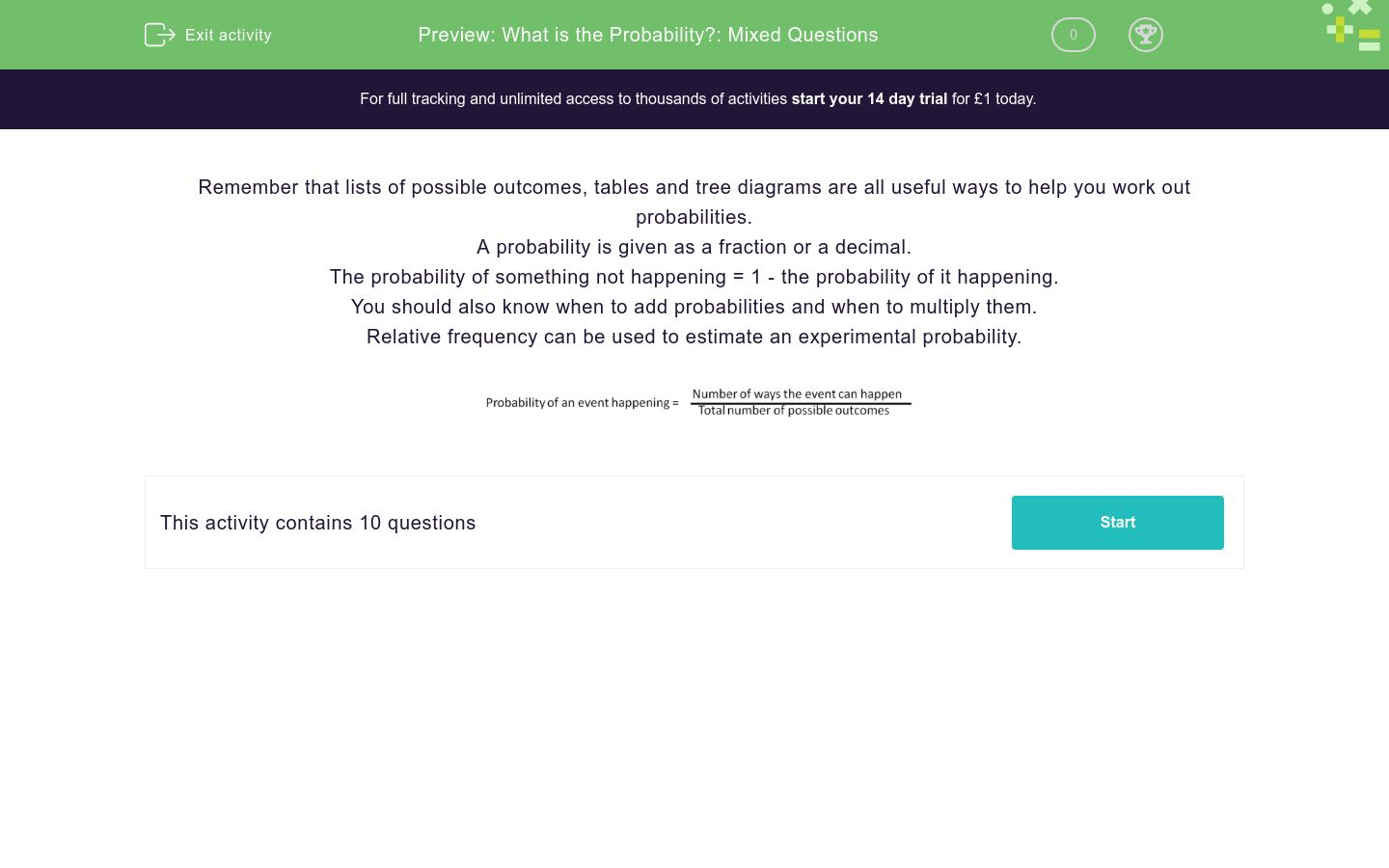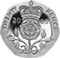# What is the Probability?: Mixed Questions

In this worksheet, students answer mixed questions on probability.Key stage:  KS 3

Curriculum topic:   Probability

Curriculum subtopic:   Calculate Theoretical Probability

Difficulty level:### QUESTION 1 of 10

Remember that lists of possible outcomes, tables and tree diagrams are all useful ways to help you work out probabilities.

A probability is given as a fraction or a decimal.

The probability of something not happening = 1 - the probability of it happening.

You should also know when to add probabilities and when to multiply them.

Relative frequency can be used to estimate an experimental probability.4 red balls, 5 yellow balls and 6 blue balls are in a bag.

A ball is chosen at random.

What is the probability that it's yellow?

4 red balls, 5 yellow balls and 6 blue balls are in a bag.

A ball is chosen at random.

What is the probability that it's not yellow?

4 red balls, 5 yellow balls and 6 blue balls are in a bag.

A ball is chosen at random.

What is the probability that it's red or yellow?

The letters of the sentence, I LOVE MATHS are placed in a bag.

A letter is chosen at random.

What is the probability that it's a vowel?

The probability that it will be sunny tomorrow is 0.25

What is the probability that it will not be sunny?

Two fair coins are thrown.

What is the probability that they will both show heads on top?

The probability that we win the pub quiz tomorrow is 0.35.

True or false? The probability that we don't win is 0.65.

True

False

The probability of passing the driving test is 0.65.

If 200 people take the test, how many would you expect to pass?

A fair die and a fair coin are thrown.What is the probability of getting a six and a tail?

A fair die is thrown and this spinner is spun.What is the probability of getting a product of 4?

• Question 1

4 red balls, 5 yellow balls and 6 blue balls are in a bag.

A ball is chosen at random.

What is the probability that it's yellow?

1/3
• Question 2

4 red balls, 5 yellow balls and 6 blue balls are in a bag.

A ball is chosen at random.

What is the probability that it's not yellow?

2/3
• Question 3

4 red balls, 5 yellow balls and 6 blue balls are in a bag.

A ball is chosen at random.

What is the probability that it's red or yellow?

3/5
• Question 4

The letters of the sentence, I LOVE MATHS are placed in a bag.

A letter is chosen at random.

What is the probability that it's a vowel?

0.4
• Question 5

The probability that it will be sunny tomorrow is 0.25

What is the probability that it will not be sunny?

0.75
• Question 6

Two fair coins are thrown.

What is the probability that they will both show heads on top?

0.25
• Question 7

The probability that we win the pub quiz tomorrow is 0.35.

True or false? The probability that we don't win is 0.65.

True
• Question 8

The probability of passing the driving test is 0.65.

If 200 people take the test, how many would you expect to pass?

130
• Question 9

A fair die and a fair coin are thrown.What is the probability of getting a six and a tail?

1/12
• Question 10

A fair die is thrown and this spinner is spun.What is the probability of getting a product of 4?

1/8
EDDIE SAYS
3/24
---- OR ----

Sign up for a £1 trial so you can track and measure your child's progress on this activity.

### What is EdPlace?

We're your National Curriculum aligned online education content provider helping each child succeed in English, maths and science from year 1 to GCSE. With an EdPlace account you’ll be able to track and measure progress, helping each child achieve their best. We build confidence and attainment by personalising each child’s learning at a level that suits them.

Get started